• 里面带有青霉素仿真数据，典型变量分析部分没有问题。
• ## python 典型变量分析

千次阅读 2018-04-18 11:17:01
典型相关分析1.典型相关分析的基本思想是首先在每组...有了这样线性组合的最大相关，则讨论两组变量之间的相关，就转化为只研究这些线性组合的最大相关，从而减少研究变量的个数.#典型变量分析得到第一典型变量 d...
典型相关分析1.典型相关分析的基本思想是首先在每组变量中找出变量的线性组合，使其具有最大相关性，然后再在每组变量中找出第二对线性组合，使其分别与第一对线性组合不相关，而第二对本身具有最大的相关性，如此继续下去，直到两组变量之间的相关性被提取完毕为止.有了这样线性组合的最大相关，则讨论两组变量之间的相关，就转化为只研究这些线性组合的最大相关，从而减少研究变量的个数.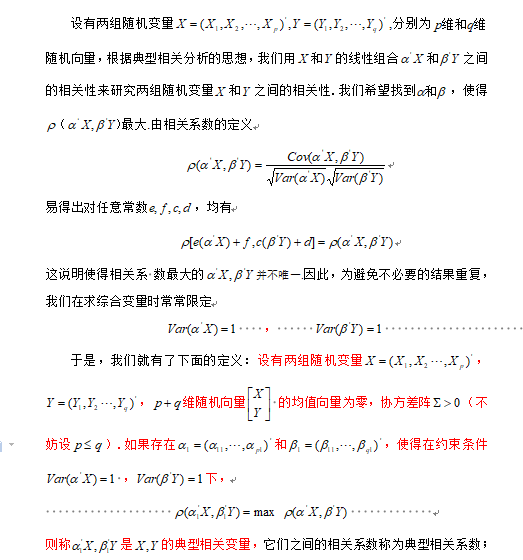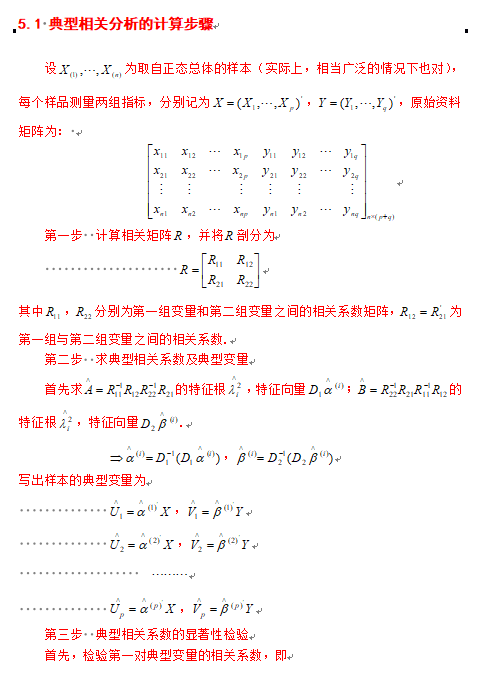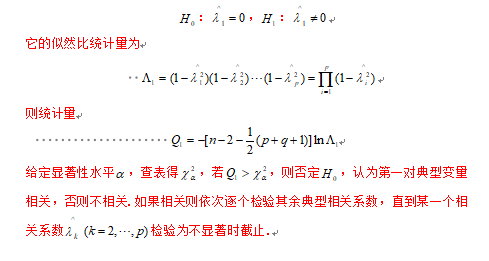#典型变量分析得到第一典型变量
def CAA(X,Y):
R= corrcoef(X,Y,rowvar=0)
m, n = shape(X)
p, q = shape(Y)
# print(m,n)
# print(p,q)

R11=[]
R22=[]
R12=[]

#计算相关系数
for i in range(n):
temp=R[i]
a=temp[0:n]
R11.append(a)

for j in range(n,n+q):
temp=R[j]
a=temp[n:n+q]
R22.append(a)

for s in range(n):
temp=R[s]
a=temp[n:n+q]
R12.append(a)

R11=matrix(R11)

R22 = matrix(R22)
R12 = matrix(R12)
R21=R12.T

#计算特征值与特征向量
M=(R11.I)*(R12)*(R22.I)*(R21)
eigVals, eigVects = linalg.eig(mat(M))  # 计算特征值和特征向量
eigValInd = sorted(eigVals,reverse=True)  # 对特征值eigVals从大到小排序
sorted_indices = np.argsort(-eigVals)#根据特征值的顺序排列相应的特征向量
topk_evecs = eigVects[:, sorted_indices[:]]
eig=sqrt(eigValInd)#计算特征值开平方
# print("特征值开方=",eig)

#计算第一对典型变量的相应的系数
k,l=shape(topk_evecs)
cout_t=[]
for i in range(l):
t=1.0/((topk_evecs[:,i].T)*R11*(topk_evecs[:,i]))
cout_t.append(t[0,0])
# print("cout_t=", cout_t)
tt=[sqrt(i) for i in cout_t]
# print("tt=",tt)

a1=tt*topk_evecs[:,0]#第一个特征值开方所对应的特征向量
p1=(1.0/eig)*(R22.I)*R21*a1#第一个特征值开方所对应的特征向量
print("特征值开方=", real(eig))
# print("a1=",a1)
# print("p1=",p1)
# print("a1.shape=", shape(a1))
# print("p1.shape=",shape(p1))

#进行显著性检验
U1 = []
V1 = []
A=1
for i in range(len(eigVals)):
A*=(1-eigVals[i])
Q1=-(m-1-1.0/2*(n+q+1))*log(A)
# print("Q1=",Q1)
if Q1>7.81:

# print("第一主成分为显著性关联")
for i in range(m):
temp1 = 0
for j in range(n):
temp1+=a1[j]*X[i,j]
# print(temp1)
U1.append(temp1[0,0])

for i in range(p):
temp2 = 0
for j in range(q):
temp2+= p1[j] * Y[i, j]
V1.append(temp2[0,0])

# print("U1,VI=",U1,V1)
else:
print("第一主成分不显著关联")

# A2=1
# for i in range(1,len(eigVals)):
# 	A2*=(1-eigVals[i])
# Q2 = -(m - 2 - 1.0 / 2 * (n + q + 1)+1.0/(sqrt(eig))) * log(A2)
# print("Q2=", Q2)
# if Q2>37.65:
# 	print("第二主成分为显著性关联")
# else :
# 	print("第二主成分不显著关联")
#
# A3=1
# for i in range(2,len(eigVals)):
# 	A3*=(1-eigVals[i])
# Q3= -(m - 3 - 1.0 / 2 * (n + q + 1)) * log(A3)
# print("Q3=", Q3)
# if Q3>26.3:
# 	print("第三主成分为显著性关联")
# else :
# 	print("第三主成分不显著关联")
return U1,V1,eig

展开全文Python
• 变量分析是数据分析中最简单的形式，其中被分析的数据只包含一个变量。因为它是一个单一的变量，它不处理原因或关系。单变量分析的主要目的是描述数据并找出其中存在的模式。 可以将变量视为数据所属的类别，比如...单变量分析是数据分析中最简单的形式，其中被分析的数据只包含一个变量。因为它是一个单一的变量，它不处理原因或关系。单变量分析的主要目的是描述数据并找出其中存在的模式。           可以将变量视为数据所属的类别，比如单变量分析中，有一个变量是“年龄”，另一个变量是“高度”等，单因素分析就不能同时观察这两个变量，也不能看它们之间的关系。          单变量数据中的发现模式有：查看平均值、模式、中位数、范围、方差、最大值、最小值、四分位数和标准偏差。此外，显示单变量数据的一些方法包括频率分布表、柱状图、直方图、频率多边形和饼状图。                    使用双变量分析来找出两个不同变量之间是否存在关系，在笛卡尔平面上（想想X和Y轴）将一个变量对另一个变量进行绘图，从而创建散点图（.plot），这样简单的事情有时可以让你了解数据试图告诉你的内容，如果数据似乎符合直线或曲线，那么这两个变量之间存在关系或相关性。例如，人们可能会选择热量摄入与体重的关系。                    多变量分析是对三个或更多变量的分析。根据你的目标，有多种方法可以执行多变量分析，这些方法中的一些包括添加树，典型相关分析，聚类分析，对应分析/多重对应分析，因子分析，广义Procrustean分析，MANOVA，多维尺度，多元回归分析，偏最小二乘回归，主成分分析/回归/ PARAFAC和冗余分析。
展开全文• 典型相关分析（Canonical correlation analysis）（二）：原始变量与典型变量之间的相关性 、典型相关系数的检验 典型相关分析（Canonical correlation analysis）（三）： 职业满意度典型相关分析案例...
典型相关分析系列博文：

典型相关分析（Canonical correlation analysis）（一）：基本思想 、复相关系数、偏相关系数

典型相关分析（Canonical correlation analysis）（二）：原始变量与典型变量之间的相关性 、典型相关系数的检验

典型相关分析（Canonical correlation analysis）（三）： 职业满意度典型相关分析案例

典型相关分析（Canonical correlation analysis）（四）： 中国城市竞争力与基础设施的相关分析

目录

1  原始变量与典型变量之间的相关性

（1）原始变量与典型变量之间的相关系数

（2）各组原始变量被典型变量所解释的方差

2  典型相关系数的检验

1．计算样本的协方差阵

2．建立整体检验 & 统计量

3．部分总体典型相关系数为零的检验

1  原始变量与典型变量之间的相关性

（1）原始变量与典型变量之间的相关系数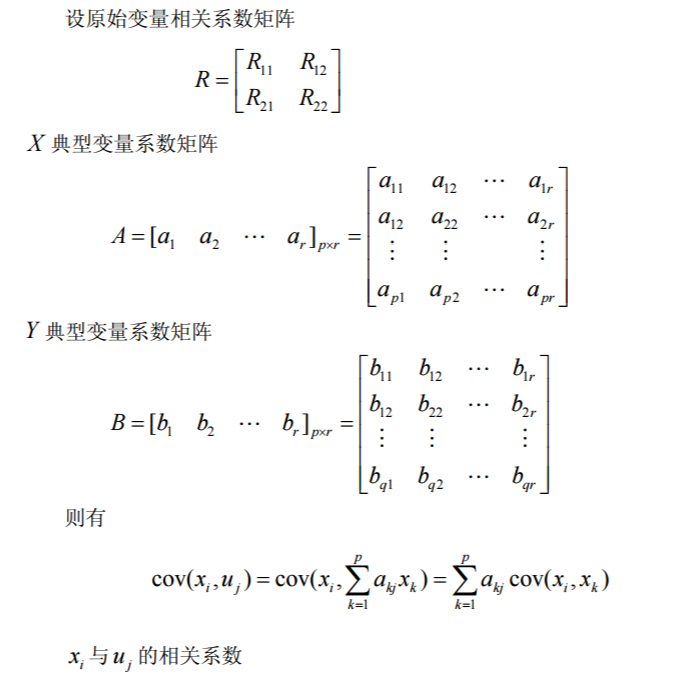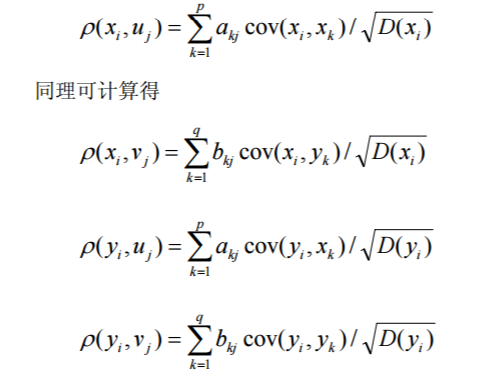（2）各组原始变量被典型变量所解释的方差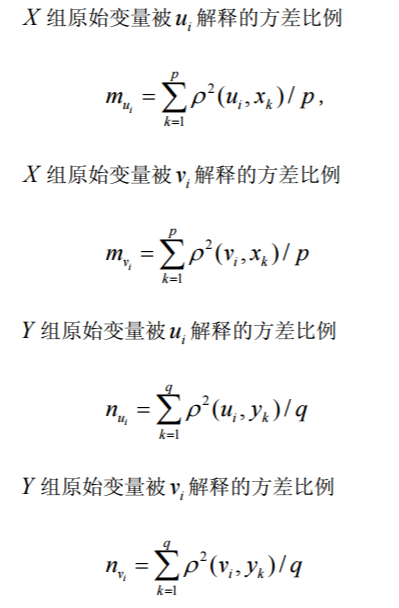2  典型相关系数的检验

在实际应用中，总体的协方差矩阵常常是未知的，类似于其他的统计分析方法，需 要从总体中抽出一个样本，根据样本对总体的协方差或相关系数矩阵进行估计，然后利 用估计得到的协方差或相关系数矩阵进行分析。由于估计中抽样误差的存在，所以估计 以后还需要进行有关的假设检验。

1．计算样本的协方差阵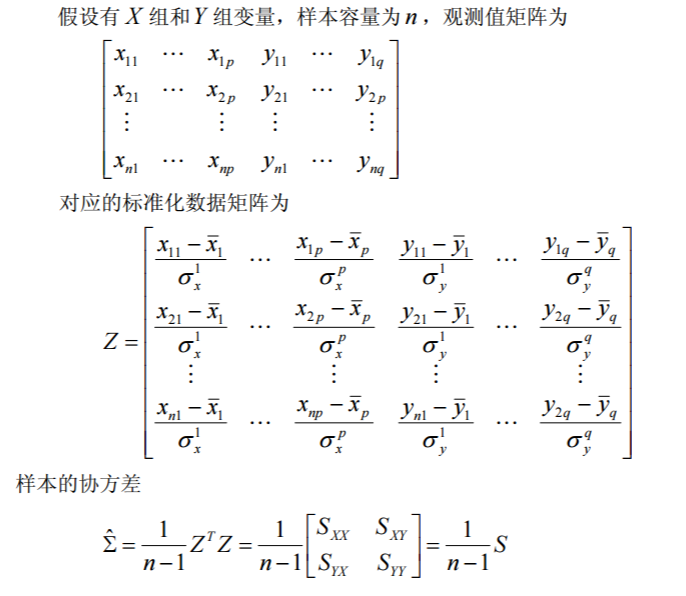2．建立整体检验 & 统计量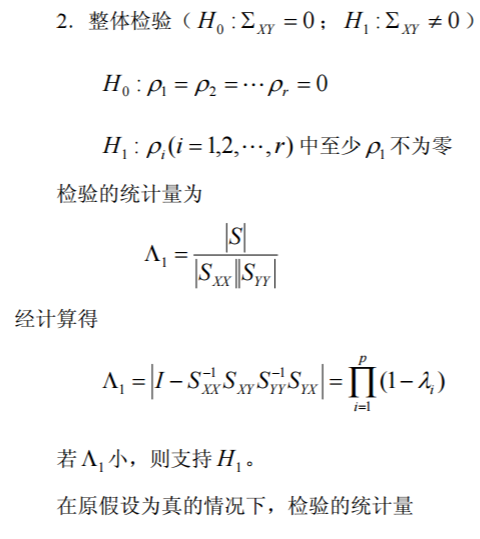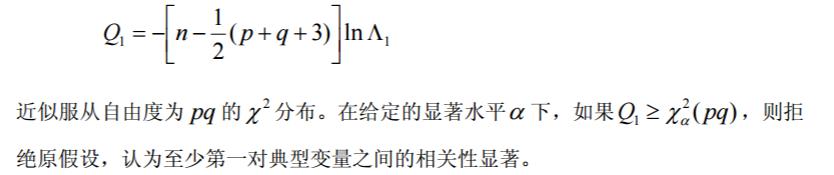3．部分总体典型相关系数为零的检验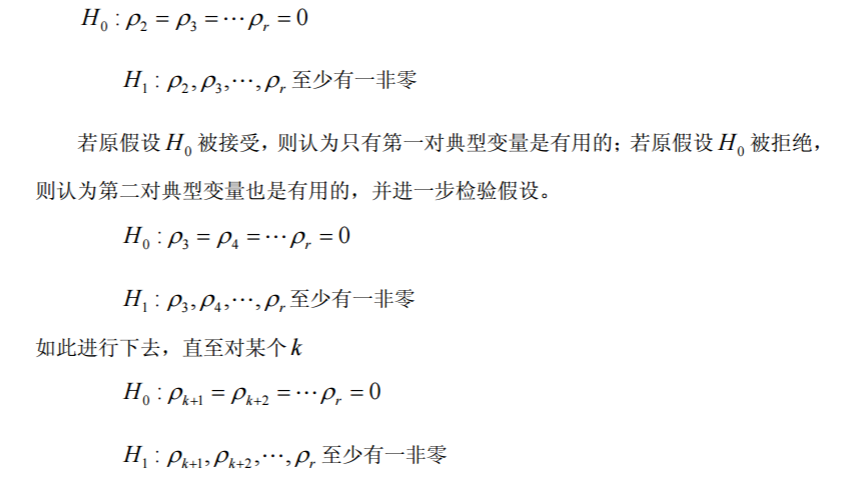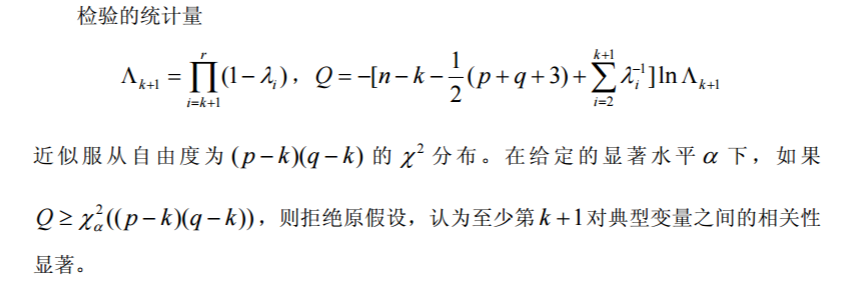典型相关分析系列博文：

典型相关分析（Canonical correlation analysis）（一）：基本思想 、复相关系数、偏相关系数

典型相关分析（Canonical correlation analysis）（二）：原始变量与典型变量之间的相关性 、典型相关系数的检验

典型相关分析（Canonical correlation analysis）（三）： 职业满意度典型相关分析案例

典型相关分析（Canonical correlation analysis）（四）： 中国城市竞争力与基础设施的相关分析


展开全文• 前言我们在分析两组变量之间的相关性时，比如X=[X1,X2,...,Xm]X=[X_1,X_2,...,X_m]和Y=[Y1,Y2,...,Yn]Y=[Y_1,Y_2,...,Y_n],最原始的方法就是直接计算X和Y的协方差矩阵，矩阵有m*n个值。有了协方差矩阵就得到了两两...
前言

我们在分析两组变量之间的相关性时，比如X=[X1,X2,...,Xm]$X=[X_1,X_2,...,X_m]$和Y=[Y1,Y2,...,Yn]$Y=[Y_1,Y_2,...,Y_n]$,最原始的方法就是直接计算X和Y的协方差矩阵，矩阵有m*n个值。有了协方差矩阵就得到了两两变量之间的相关性，比如cij$c_ij$就是变量Xi$X_i$和Yj$Y_j$的相关性。

这种分析方法只考虑了cij$c_ij$变量Xi$X_i$和Yj$Y_j$变量单个维度之间的相关性，没有考虑X和Y内部之间的相关性。另外协方差矩阵的形式也比较繁琐。

典型相关分析

采用类似主成分分析的思想，分别找出两组变量各自的线性组合，然后讨论线性组合之间的相关关心。

分别在两组变量中找出第一对线性组合，使他们具有最大相关性；接着再分别在两组变量中找出第二对线性组合，使他们具有第二大的相关性，并且它们分别于本组内的第一线性组合不相关。以此类推找出其他线性组合，如下面提取两对线性组合，其中 u1u2$u_1 u_2$互相独立，v1v2$v_1 v_2$互相独立，u1v1$u_1 v_1$相关。

⎧⎩⎨⎪⎪u1=a11x1+a12x2+...+a1mxmv1=b11y1+b12y2+...+b1nyn
\left\{\begin{matrix}
u_1 = a_{11}x_1 + a_{12}x_2 + ...+ a_{1m}x_m& \\
\\
v_1 = b_{11}y_1 + b_{12}y_2 +...+ b_{1n}y_n&
\end{matrix}\right.

⎧⎩⎨⎪⎪u2=a21x1+a22x2+...+a2mxmv2=b21y1+b22y2+...+b2nyn
\left\{\begin{matrix}
u_2 = a_{21}x_1 + a_{22}x_2 + ...+ a_{2m}x_m& \\
\\
v_2 = b_{21}y_1 + b_{22}y_2 +...+ b_{2n}y_n&
\end{matrix}\right.

假设提取了r次，则最后提取了r组变量，
U=(u1,...,ur)TV=(v1,...,vr)T
U = (u_1,...,u_r)^T \\
V = (v_1,...,v_r)^T

则实现通过线性组合来表示原变量，并且用他们之间的相关性来表示原变量的相关性。

本质是什么

典型相关分析的本质是从两组变量中选取若干个有代表性的变量线性组合，用这些线性组合的相关性来表示原来两组变量的相关性。

求解线性组合

以第一组线性组合为例，这里要求解的就是包含的系数。

⎧⎩⎨⎪⎪u1=a11x1+a12x2+...+a1mxmv1=b11y1+b12y2+...+b1nyn
\left\{\begin{matrix}
u_1 = a_{11}x_1 + a_{12}x_2 + ...+ a_{1m}x_m& \\
\\
v_1 = b_{11}y_1 + b_{12}y_2 +...+ b_{1n}y_n&
\end{matrix}\right.

矩阵形式为，

⎧⎩⎨⎪⎪u1=aT1Xv1=bT1Y
\left\{\begin{matrix}
u_1 = a_1^TX& \\
\\
v_1 = b_1^TY&
\end{matrix}\right.

只考虑方差为1时的线性函数，这时其实就存在一个约束条件，D(u1)=D(v1)=1$D(u_1) = D(v_1) = 1$，我们求常向量aT1$a_1^T$和bT1$b_1^T$，使得相关系数ρ(u1,v1)$\rho(u_1,v_1)$达到最大。

方差分别为，
D(u1)=D(aT1X)=aT1Cov(X,X)a1D(v1)=D(bT1Y)=bT1Cov(Y,Y)b1
D(u_1) = D(a_1^TX) = a_1^TCov(X,X)a_1\\
D(v_1) = D(b_1^TY) = b_1^TCov(Y,Y)b_1

则u1v1$u_1 v_1$的协方差为，

Cov(u1,v1)=aT1Cov(X,Y)b1Cov(u_1,v_1) = a_1^TCov(X,Y)b_1

另外我们还有，
Cov(X,X)=∑11Cov(Y,Y)=∑22Cov(X,Y)=∑12
Cov(X,X) = \sum_{11}\\
Cov(Y,Y) = \sum_{22}\\
Cov(X,Y) = \sum_{12}

两者的相关系数为，

Corr(u1,v1)=Cov(u1,v1)D(u1)−−−−−√D(v1)−−−−−√=aT1∑12b1aT1∑11a1−−−−−−−−√bT1∑22b1−−−−−−−−√
Corr(u_1,v_1) = \frac{Cov(u_1,v_1)}{\sqrt{D(u_1)} \sqrt{D(v_1)}} = \frac{a_1^T\sum_{12}b_1}{\sqrt{a_1^T\sum_{11}a_1} \sqrt{b_1^T\sum_{22}b_1}}

我们要做的是寻找a1b1$a_1 b_1$使相关系数最大，同时要考虑前面说到的约束条件D(u1)=D(v1)=1$D(u_1) = D(v_1) = 1$，它能防止结果重复出现，则相关系数变为，

Corr(u1,v1)=aT1∑12b1Corr(u_1,v_1) =a_1^T\sum_{12}b_1

求条件极值一般可以引入拉格朗日乘数来求极大值，求解出aT1bT1$a_1^T b_1^T$。

对于其他组的线性组合也同样根据上述过程得到解。

线性组合数量

根据线性组合相关性大小不断提取线性组合，最大的为第一组，次大的为第二组，以此类推直到 r

怎么分析

比如第一组线性组合中，可以计算两组变量与u1和v1的相关系数，如果某若干个变量与u1相关系数较大，则u1代表该若干变量的总指标，可以自己根据变量取个额外的名称。而同样如果v1和其他若干变量的相关系数较大的话则v1可代表该若干变量。

接着如果u1和v1的相关系数较大的话则说明u1代表的若干变量与v1代表的若干变量是关系密切的。对于其他线性组合也一样，先找出u2和v2相关的若干变量，然后再看u2与v2之间的相关系数，判断其是否相关。

=============广告时间===============

公众号的菜单已分为“分布式”、“机器学习”、“深度学习”、“NLP”、“Java深度”、“Java并发核心”、“JDK源码”、“Tomcat内核”等，可能有一款适合你的胃口。

鄙人的新书《Tomcat内核设计剖析》已经在京东销售了，有需要的朋友可以购买。感谢各位朋友。

为什么写《Tomcat内核设计剖析》

=========================

欢迎关注：展开全文机器学习 统计学
• 1.常见的几种相关两个连续变量之间的线性相关关系可以采用“简单相关分析”，计算Pearson积差相关分析或Spearman秩相关分析。具体查看：两个变量间的线性相关关系(SPSS：线性相关分析)一个连续变量与一组(多个)连续...
• 相关分析是研究两两变量间关系的方法，在现实生活中，变量间的关系往往更加复杂。比如，要考察多个变量与多个变量（即两组...它是借助主成分分析思想，从两组变量中提取出一个或少数几个综合变量（即典型变量），从...
• 周末好呀！我是一只正在迭代的分析狮天天。...统计学连续变量(定距)、分类变量(定序、定类)相关分析、各种相关系数SPSS操作分析-描述统计-交叉表分析-相关图形-图形构建器，散点图、条形图发现事物之间的关...
• 做一下推广，如果研究一个变量和多个随机变量之间的线性相关关系时，提出了全相关系数（或者复相关系数）的概念。然后，在1936年，有个叫做hotelling的数学家，又进一步做了推广，研究 多个随机变量和多个随机变量...
• 点击“蓝字”关注我们典型相关分析一、典型相关分析概述1.1基本思想在一元统计分析中，两个随机变量X，Y之间的线性相关关系可用简单相关系数描述；一个随机变量Y和一组随机变量X之间的线性相关关系可用复相关系数...
• 我们已经知道，两个随机变量间的相关关系可以用简单相关系数表示，一个随机变量和多个随机变量的相关关系可以用复相关系数表示，而如果需要研究多个随机变量和多个随机变量间的相关关系，则需要使用典型相关分析。...
• 在社会科学研究中，主要的多变量分析方法包括多变量方差分析(Multivariate analysis of variance，MANOVA)、主成分分析(Principal component analysis)、因子分析(Factor analysis)、典型相关(Canonical correlation...
• 4、SPSSAU输出结果此表格展示出典型变量的提取情况，上表中共显示有5个典型变量被提取出来，在进行F检验时显示，其中仅2个典型变量是呈现出0.01水平的显著性，因此，最终应该以两个典型变量为准进行后续研究。...
• 方法简介 在相关分析一章中我们主要研究的是两个变量间的相关顶多调整其他因素的作用而已 如果要研究一个变量和一组变量间的相关则可以使用多元线性回归方程的复相关系数就 是我们要的东西同时偏相关系数还可以描述...
• 1 关键点：典型相关分析典型相关分析是用于分析两组随机变量之间的相关程度的一种统计方法，它能够有效地揭示两组随机变量之间的相互(线性依赖)关系例如 研究生入学考试成绩与本科阶段一些主要课程成绩的相关性将...
• 典型相关分析在对经济问题的研究和管理研究中，不仅经常需要考察两个变量之间的相关程度，而且还经常需要考察多个变量与多个变量之间即两组变量之间的相关性。典型相关分析就是测度两组变量之间相关程度的一种多元...
• ## 典型相关分析

千次阅读 2019-06-11 14:27:12
典型相关分析函数：[a,b,r,u,v,stats] = cononcorr(x,y): param: 　x：原始变量x矩阵，每列一个自变量指标，第i列是 xi 的样本值 　y：原始变量y矩阵，每列一个因变量指标，第j列是 yj 的样本值 return: 　a：...
• 在社会科学研究中，主要的多变量分析方法包括多变量方差分析（Multivariate analysis of variance，MANOVA）、主成分分析（Principal component analysis）、因子分析（Factor analysis）、典型相关（Canonical ...
• 在社会科学研究中，主要的多变量分析方法包括多变量方差分析(Multivariate analysis of variance，MANOVA)、主成分分析(Principal component analysis)、因子分析(Factor analysis)、典型相关(Canonical correlation...
• 结果整理结果整理如下表所示，这里只取数据的绝对值。从表中数据可得到下列结论：1.本例中的两对变量对X和Y，因为X有5个观察变量，Y有4个观察变量，所以...2.X的5个观察变量中，观察变量x5对第1典型变量U1的关系最紧...
• Canonical Correlation Analysis 典型相关分析介绍定义典型相关分析(Canonical Correlation Analysis)12利用综合变量对之间的相关关系来反映两组指标之间的整体相关性的多元统计分析方法。输入：两个随机变量组\(X =...
• 多重对应分析在超过两个以上定类变量时有时候非常有效，当然首先我们要理解并思考，如果只有三个或有限的几个变量完全可以通过数据变换和交互表变量重组可以转换成两个定类变量，这时候就可以用简单对应分析了。...
• 典型相关分析（Canonical correlation analysis）（二）：原始变量与典型变量之间的相关性 、典型相关系数的检验 典型相关分析（Canonical correlation analysis）（三）： 职业满意度典型相关分......